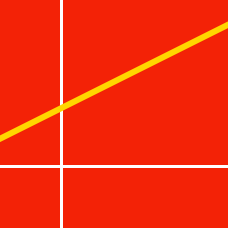Geometry

# Intercepts of a Line

What is the x-intercept of the line $y = 5x - 40$?

What is the $y$-intercept of the line with slope $m = 1$ that passes through the point $(12,\ 36)$?

Let $y=-6x+42$ be the equation of a line. What is the sum of the $x$-intercept and $y$-intercept?

If a line has $x$-intercept $A$ and $y$-intercept $B,$ and the midpoint of line segment $AB$ is $P=(8, 11)$, what is the equation the line?

What is the $y$-intercept of the line $\sqrt{3}x-y+34=0?$

×